Courses

# Chapter 7 Entropy - Thermodynamics, Mechanical Engineering Mechanical Engineering Notes | EduRev

## Mechanical Engineering: Chapter 7 Entropy - Thermodynamics, Mechanical Engineering Mechanical Engineering Notes | EduRev

The document Chapter 7 Entropy - Thermodynamics, Mechanical Engineering Mechanical Engineering Notes | EduRev is a part of the Mechanical Engineering Course Mechanical Engineering SSC JE (Technical).
All you need of Mechanical Engineering at this link: Mechanical Engineering

ENTROPY

• Two reversible adiabatic paths cannot intersects each other which violates the Kelvin - Plank's statement.
• Clausius Theorem

Any reversible path may be substituted by a reversible zigzag path between the same end states, consisting of a reversible adiabatic followed by a reversible isotherm and then by reversible adiabatic such that the heat transferred during the isothermal process is the same as that transfered during the original process.

• The cyclic integral of  (dQ/T)  for a reversible cycle is equal to zero.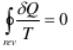• Entropy:

The entropy of a system is a thermodynamic property which is a measure of the degree of molecular disorder existing in the system. It describes the randomness or uncertainity of the system, It ios a function of a quantity of heat which shows the possibility of conversion of heat into work. Thus, for maximum entropy, there is minimum availability for conversion into work and for minimum entropy there is a maximum availability for conversion into work.

Characteristics:

1.  It increases when heat is supplied irrespective of the fact whether temperature changes or not.
2.  It dectreases when heat is removed whether the temperature changes or not.
3.  It remains unchanged in all adiabatic reversible processes.
4.  The increase in entropy is small when heat is added at a high temperature and is greater when heat addition is made at a lower temperature.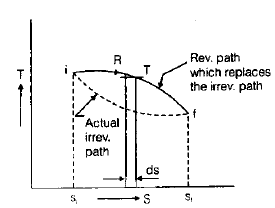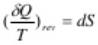=> dS = 0 (∵ dQ = 0)
∴ S = constant

Thus a reversible adiabatic process is an isentropic process.

•   For reversible isothermal process

At a temperature, To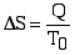CLAUSIS' INEQUALITY

The Clausius theorem (1855) states that a system (heat engine or heat pump) exchanging heat with external reservoirs and undergoing a cyclic process, is one that ultimately returns a system to its original state,

∮                                                              ⁡                                            δ              Q                                      T                              s                u                r                r                                                    ≤        0        ,              {\displaystyle \oint {\frac {\delta Q}{T_{surr}}}\leq 0,}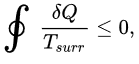where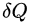δ        Q              {\displaystyle \delta Q}    is the infinitesimal amount of heat absorbed by the system from the reservoir and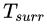T                      s            u            r            r                                {\displaystyle T_{surr}}    is the temperature of the external reservoir (surroundings) at a particular instant in time.

Remember:-

Equality sign holds good for a reversible process and the inequality sign for an irreversible process.
Entropy principle:

For an isolated reversible system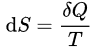For an isolated irreversible system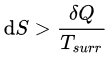The total entropy of an isolated system can never decrease over time, and is constant if and only if all processes are reversible. Isolated systems spontaneously evolve towards thermodynamic equilibrium, the state with maximum entropy.
• It is also a statement of second law of thermodynamics.
• Entropy increase of the isolated system is a measure of the extent of irreversibility
of the process under gone by the system.
• When the system is at equilibrium, any conceivable change in entropy would be
zero.
Application of entropy principle
• Transfer of heat through a finite Temperature difference.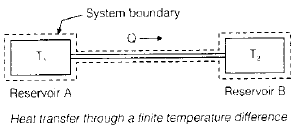Mixing of two fluids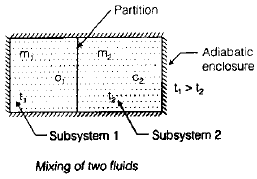Final temp (tf) =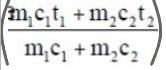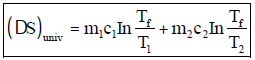Maximum work obtainable from two finite identical bodies at T1 and T2.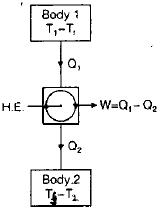Final temperature of the two bodies (Tf) =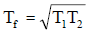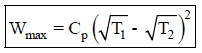• The final temperature of two bodies initally at T1 & T2, can range from (T1 + T2)/2 with no delivery of work to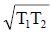with maximum delivery of work.

• Maximum work obtained with finite body & TER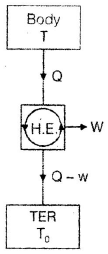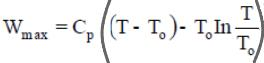T = temp. of body
To = temp. of TER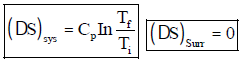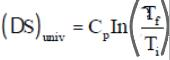• Adiabatic process vs Isentropic process
• If isentropic process is reversible, it must be adibatic.
• If isentropic process is adiabatic, it must be reversible.
• An adiabatic process may not be isentropic because entropy may change due to friction, internal irreversibility etc.
• If the adiabatic process is reversible, it must be isentropic.
•  Change in entropy of a system is due to heat transfer and internal irreversibility (entropy generation.)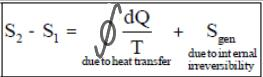• Entropy is a point function but entropy generation is a path function.
 Equation Holds good for dQ = dE+ dW Reversible, Irreversible, any system dQ = dU + dW Reversible, Irreversible, Closed System. dQ = dU + pdV Reversible, Closed system dQ = TdS Reversible TdS = dU + PdV Reversible, Irreversible, Closed system TdS = dH – Vdp Reversible, Irreversible, Closed system
• Entropy change of a system (solid, liquid ) when its temperature changes from T1 to T2.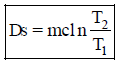c : specific heat for solid, liquid

The document Chapter 7 Entropy - Thermodynamics, Mechanical Engineering Mechanical Engineering Notes | EduRev is a part of the Mechanical Engineering Course Mechanical Engineering SSC JE (Technical).
All you need of Mechanical Engineering at this link: Mechanical EngineeringUse Code STAYHOME200 and get INR 200 additional OFF Use Coupon Code
All Tests, Videos & Notes of Mechanical Engineering: Mechanical Engineering

### Top Courses for Mechanical Engineering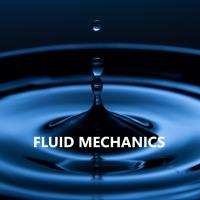## Mechanical Engineering SSC JE (Technical)

83 docs|53 tests

### Top Courses for Mechanical EngineeringTrack your progress, build streaks, highlight & save important lessons and more!

,

,

,

,

,

,

,

,

,

,

,

,

,

,

,

,

,

,

,

,

,

,

,

,

;